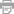# One Letra

## Exxplorer

### A Recipe For Power

Letra de One
[Hary Nilsson cover]

One is the loneliest number
That you'll ever do
Two can be as bad as one
It's the loneliest number
Since the number one

You'll ever know
You'll ever know

'Cause one is the loneliest number
That you'll ever do
One is the loneliest number or would it be two

It's just no good anymore
Since you went away
Now I spend my time
Just making rhymes of yesterday

One is the loneliest number
One is the loneliest
One is the loneliest number
That you'll ever do
One is the loneliest
One is the loneliest
One is the loneliest number
That you'll ever do

It's just no good anymore
Since you went away

Number
One is the loneliest number
One is the loneliest number
One is the loneliest number
One is the loneliest number
That you'll ever do
One is the loneliest number
One is the loneliest number
One is the loneliest number
One is the loneliest number
That you'll ever do
I'm so lonely
I'm so lonely
I'm so lonely
•Imprimir Letra